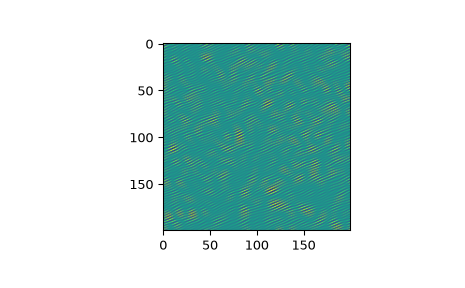# scipy.fft.ifftn¶

scipy.fft.ifftn(x, s=None, axes=None, norm=None, overwrite_x=False, workers=None, *, plan=None)[source]

Compute the N-D inverse discrete Fourier Transform.

This function computes the inverse of the N-D discrete Fourier Transform over any number of axes in an M-D array by means of the Fast Fourier Transform (FFT). In other words, ifftn(fftn(x)) == x to within numerical accuracy.

The input, analogously to ifft, should be ordered in the same way as is returned by fftn, i.e., it should have the term for zero frequency in all axes in the low-order corner, the positive frequency terms in the first half of all axes, the term for the Nyquist frequency in the middle of all axes and the negative frequency terms in the second half of all axes, in order of decreasingly negative frequency.

Parameters
xarray_like

Input array, can be complex.

ssequence of ints, optional

Shape (length of each transformed axis) of the output (s refers to axis 0, s to axis 1, etc.). This corresponds to n for ifft(x, n). Along any axis, if the given shape is smaller than that of the input, the input is cropped. If it is larger, the input is padded with zeros. if s is not given, the shape of the input along the axes specified by axes is used. See notes for issue on ifft zero padding.

axessequence of ints, optional

Axes over which to compute the IFFT. If not given, the last len(s) axes are used, or all axes if s is also not specified.

norm{“backward”, “ortho”, “forward”}, optional

Normalization mode (see fft). Default is “backward”.

overwrite_xbool, optional

If True, the contents of x can be destroyed; the default is False. See fft for more details.

workersint, optional

Maximum number of workers to use for parallel computation. If negative, the value wraps around from os.cpu_count(). See fft for more details.

plan: object, optional

This argument is reserved for passing in a precomputed plan provided by downstream FFT vendors. It is currently not used in SciPy.

New in version 1.5.0.

Returns
outcomplex ndarray

The truncated or zero-padded input, transformed along the axes indicated by axes, or by a combination of s or x, as explained in the parameters section above.

Raises
ValueError

If s and axes have different length.

IndexError

If an element of axes is larger than than the number of axes of x.

fftn

The forward N-D FFT, of which ifftn is the inverse.

ifft

The 1-D inverse FFT.

ifft2

The 2-D inverse FFT.

ifftshift

Undoes fftshift, shifts zero-frequency terms to beginning of array.

Notes

Zero-padding, analogously with ifft, is performed by appending zeros to the input along the specified dimension. Although this is the common approach, it might lead to surprising results. If another form of zero padding is desired, it must be performed before ifftn is called.

Examples

>>> import scipy.fft
>>> x = np.eye(4)
>>> scipy.fft.ifftn(scipy.fft.fftn(x, axes=(0,)), axes=(1,))
array([[1.+0.j,  0.+0.j,  0.+0.j,  0.+0.j], # may vary
[0.+0.j,  1.+0.j,  0.+0.j,  0.+0.j],
[0.+0.j,  0.+0.j,  1.+0.j,  0.+0.j],
[0.+0.j,  0.+0.j,  0.+0.j,  1.+0.j]])


Create and plot an image with band-limited frequency content:

>>> import matplotlib.pyplot as plt
>>> n = np.zeros((200,200), dtype=complex)
>>> n[60:80, 20:40] = np.exp(1j*np.random.uniform(0, 2*np.pi, (20, 20)))
>>> im = scipy.fft.ifftn(n).real
>>> plt.imshow(im)
<matplotlib.image.AxesImage object at 0x...>
>>> plt.show()scipy.fft.fftn

scipy.fft.rfft